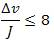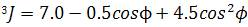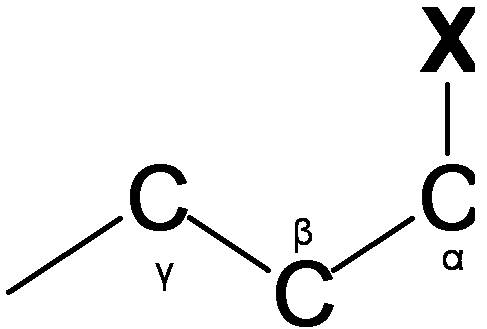# NMR: Interpretation

NMR interpretation plays a pivotal role in molecular identifications. As interpreting NMR spectra, the structure of an unknown compound, as well as known structures, can be assigned by several factors such as chemical shift, spin multiplicity, coupling constants, and integration.

## Introduction

Here is a strategy for solving structure:

1. Molecular formula is determined by chemical analysis such as elementary analysis
2. Double-bond equivalent (also known as Degree of Unsaturation) is calculated by a simple equation to estimate the number of the multiple bonds and rings. It assumes that oxygen (O) and sulfur (S) are ignored and halogen (Cl, Br) and nitrogen is replaced by CH. The resulting empirical formula is CaHb1. Structure fragmentation is determined by chemical shift, spin multiplicity, integral (peak area), and coupling constant(1J, 2J)
2. Molecular skeleton is built up using 2-dimensional NMR spectroscopy.
3. Relative configuration is predicted by coupling constant (3J).

This Module focuses on the most important 1H and 13C NMR spectra to find out structure even though there are various kinds of NMR spectra such as 14N, 19F, and31P. NMR spectrum shows that x- axis is chemical shift in ppm. It also contains integral areas, splitting pattern, and coupling constant.

## 1H NMR

### Chemical Shift

Chemical shift is associated with the Larmor frequency of a nuclear spin to its chemical environment. Tetramethylsilan[TMS;(CH3)4Si] is generally used for standard to determine chemical shift of compounds: δTMS=0ppm. In other words, frequencies for chemicals are measured for a 1H or 13C nucleus of a sample from the 1H or 13C resonance of TMS. It is important to understand trend of chemical shift in terms of NMR interpretation. The proton NMR chemical shift is affect by nearness to electronegative atoms (O, N, halogen.) and unsaturated groups (C=C,C=O, aromatic). Electronegative groups move to the down field (left; increase in ppm). Unsaturated groups shift to downfield (left) when affecting nucleus is in the plane of the unsaturation, but reverse shift takes place in the regions above and below this plane. 1H chemical shift play a role in identifying many functional groups. Figure 1. indicates important example to figure out the functional groups.Figure 1. 1H chemical shift ranges for organic compound

### Chemical equivalence

Protons with Chemical equivalence has the same chemical shift due to symmetry within molecule ($$CH_3COCH_3$$) or fast rotation around single bond (-CH3; methyl groups).

## Spin-Spin Splitting

Spin-Spin splitting means that an absorbing peak is split by more than one “neighbor” proton. Splitting signals are separated to J Hz, where is called the coupling constant. The spitting is a very essential part to obtain exact information about the number of the neighboring protons. The maximum of distance for splitting is three bonds. Chemical equivalent protons do not result in spin-spin splitting. When a proton splits, the proton’s chemical shift is determined in the center of the splitting lines.

#### Spin Multiplicity (Splitting pattern)

Spin Multiplicity plays a role in determining the number of neighboring protons. Here is a multiplicity rules: In case of $$A_mB_n$$ system, the multiplicity rule is that Nuclei of $$B$$ element produce a splitting the $$A$$ signal into $$nB+1$$ lines. The general formula which applies to all nuclei is $$2_nI+1$$, where $$I$$ is the spin quantum number of the coupled element. The relative intensities of the each lines are given by the coefficients of the Pascal’s triangle (Fig.2).Fig 2. Pascal's triangle

#### First-order splitting pattern

The chemical shift difference in Hertz between coupled protons in Hertz is much larger than the $$J$$ coupling constant:

$\dfrac{\Delta \nu }{J} \ge 8$

Where $$\Delta \nu$$ is the difference of chemical shift. In other word, the proton is only coupled to other protons that are far away in chemical shift. The spectrum is called first-order spectrum. The splitting pattern depends on the magnetic field. The second-order splitting at the lower field can be resolved into first-order splitting pattern at the high field. The first-order splitting pattern is allowed to multiplicity rule (N+1) and Pascal’s triangle to determine splitting pattern and intensity distribution.

Example 1

The note is that structure system is A3M2X2. Ha and Hx has the triplet pattern by Hm because of N+1 rule. The signal of Hm is split into six peaks by Hx and Ha (Fig.3) The First order pattern easily is predicted due to separation with equal splitting pattern.Figure 3: An example of splitting pattern

#### High-order splitting pattern

High-order splitting pattern takes place when chemical shift difference in hertz is much less or the same that order of magnitude as the j coupling.The second order pattern is observed as leaning of a classical pattern.: the inner peaks are taller and the outer peaks are shorter in case of AB system(Fig 4). This is called the “roof effect.”Fig 4. a) first-order pattern and b) second-order pattern of AB system

Here is other system as an example: A2B2 (Fig 5). The two triplet incline toward each other. Outer lines of the triplet are less than 1in relative area and the inner lines are more than 1. The center lines have relative area 2.Fig 5. a) first-order pattern and b) second-order pattern of A2B2 system

#### Coupling constant (J Value)

Coupling constant is the strength of the spin-spin splitting interaction and the distance between the split lines. The value of distance is equal or different depending on the coupled nuclei. The coupling constants reflect the bonding environments of the coupled nuclei. Coupling constant is classified by the number of bonds:

##### Geminal proton-proton coupling (2JHH)

Germinal coupling generates through two bonds (Fig 6). Two proton having geminal coupling are not chemically equivalent. This coupling ranges from -20 to 40 Hz. 2JHHdepends on hybridization of carbon atom and the bond angle and the substituent such as electronegative atoms. When S-character is increased, Geminal coupling constant is increased : 2Jsp1>2Jsp2>2Jsp3 The bond angle(HCH) gives rise to change 2JHH value and depend on the strain of the ring in the cyclic systems. Geminal coupling constant determines ring size. When bond angle is decreased, ring size is decreased so that geminal coupling constant is more positive. If a atom is replace to an electronegative atom, Geminal coupling constant move to positive value.Fig 6. Geminal coupling

##### Vicinal proton-proton coupling (3JHH)

Vicinal coupling occurs though three bonds (Fig 7.). The Vicinal coupling is the most useful information of dihedral angle, leading to stereochemistry and conformation of molecules. Vicinal coupling constant always has the positive value and is affected by the dihedral angle (?;HCCH), the valence angle (?; HCC), the bond length of carbon-carbon, and the effects of electronegative atoms. Vicinal coupling constant depending on the dihedral angle (Fig 8) is given by the Karplus equation.When ? is the 90o, vicinal coupling constant is zero. In addition, vicinal coupling constant ranges from 8 to 10 Hz at the and ?=180o, where ?=0o and ?=180o means that the coupled protons have cis and trans configuration, respectively.Fig 7. Vicinal coupling

The valence angle(?;Fig 8) also causes change of 3JHH value. Valence angle is related with ring size. Typically, when the valence angle decreases, the coupling constant reduces. The distance between the carbons atoms gives influences to vicinal coupling constantFig8. a) Dihedral angle and b) valence angle

The coupling constant increases with the decrease of bond length. Electronegative atoms affect vicinal coupling constants so that electronegative atoms decrease the vicinal coupling constants.

#### Integral

Integral is referred to integrated peak area of 1H signals. The intensity is directly proportionally to the number of hydrogen.

## 13C NMR

#### Chemical ShiftThe Carbon NMR is used for determining functional groups using characteristic shift values. 13C chemical shift is affect by electronegative effect and steric effect. If an H atoms in an alkane is replace by substituent X, electronegative atoms (O, N, halogen), ?-carbon and ?-carbon shift to downfield (left; increase in ppm) while ?-carbon shifts to upfield. The steric effect is observed in acyclic and clyclic system, which leads to downshifted chemical shifts. Figure 9 shows typical 13C chemical shift regions of the major chemical class.Figure 9: 13C Chemical shift range for organic compound

#### Spin-Spin splitting

Comparing the 1H NMR, there is a big difference thing in the 13C NMR. The 13C-13Cspin-spin splitting rarely exit between adjacent carbons because 13C is naturally lower abundant (1.1%)

• 13C-1H Spin coupling: 13C-1H Spin coupling provides useful information about the number of protons attached a carbon atom. In case of one bond coupling (1JCH), -CH, -CH2, and CH3 have respectively doublet, triplet, quartets for the 13C resonances in the spectrum. However, 13C-1H Spin coupling has an disadvantage for 13C spectrum interpretation. 13C-1H Spin coupling is hard to analyze and reveal structure due to a forest of overlapping peaks that result from 100% abundance of 1H.
• Decoupling: Decoupling is the process of removing 13C-1H coupling interaction to simplify a spectrum and identify which pair of nuclei is involved in the J coupling. The decoupling 13C spectra shows only one peak(singlet) for each unique carbon in the molecule(Fig 10.). Decoupling is performed by irradiating at the frequency of one proton with continuous low-power RF.Fig 10. Decoupling in the 13C NMR

• Distortions enhancement by polarization transfer (DEPT): DEPT is used for distinguishing between a CH3 group, a CH2 group, and a CH group. The proton pulse is set at 45o, 90o, or 135o in the three separate experiments. The different pulses depend on the number of protons attached to a carbon atom. Fig 11. is an example about DEPT spectrum.Fig 11. DEPT spectrum of n-isobutlybutrate

### 2-dimensional NMR spectroscopy (COSY)

COSY stands for COrrelation SpectroscopY. COSY spectrum is more useful information about what is being correlated.

#### 1H-1H COSY (COrrelation SpectroscopY)

1H-1H COSY is used for clearly indicate correlation with coupled protons. A point of entry into a COSY spectrum is one of the keys to predict information from it successfully. Relation of Coupling protons is determined by cross peaks(correlation peaks) and in the COSY spectrum. In other words, Diagonal peaks by lines are coupled to each other. Figure 12 indicates that there are correlation peaks between proton H1 and H2 as well as between H2 and H4. This means the H2 coupled to H1 and H4.Fig 12. 1H-1H COSY spectrum

#### 1H-13C COSY (HETCOR)

1H-13C COSY is the heteronuclear correlation spectroscopy. The HETCOR spectrum is correlated 13C nuclei with directly attached protons. 1H-13C coupling is one bond. The cross peaks mean correlation between a proton and a carbon (Fig 13). If a line does not have cross peak, this means that this carbon atoms has no attached proton (e.g. a quaternary carbon atom)Fig 13. 1H-13C COSY spectrum

## References

1. Balc*, M., Basic p1 sH- and p13 sC-NMR spectroscopy. 1st ed.; Elsevier: Amsterdam ; Boston, 2005; p xii, 427.
2. Breitmaier, E., Structure elucidation by NMR in organic chemistry : a practical guide. 3rd rev. ed.; Wiley: Chichester, West Sussex, England, 2002; p xii, 258.
3. Jacobsen, N. E., NMR spectroscopy explained : simplified theory, applications and examples for organic chemistry and structural biology. Wiley-Interscience: Hoboken, N.J., 2007; p xv, 668.
4. Silverstein, R. M.; Webster, F. X., Spectrometric identification of organic compounds. 6th ed.; Wiley: New York, 1998; p xiv, 482.